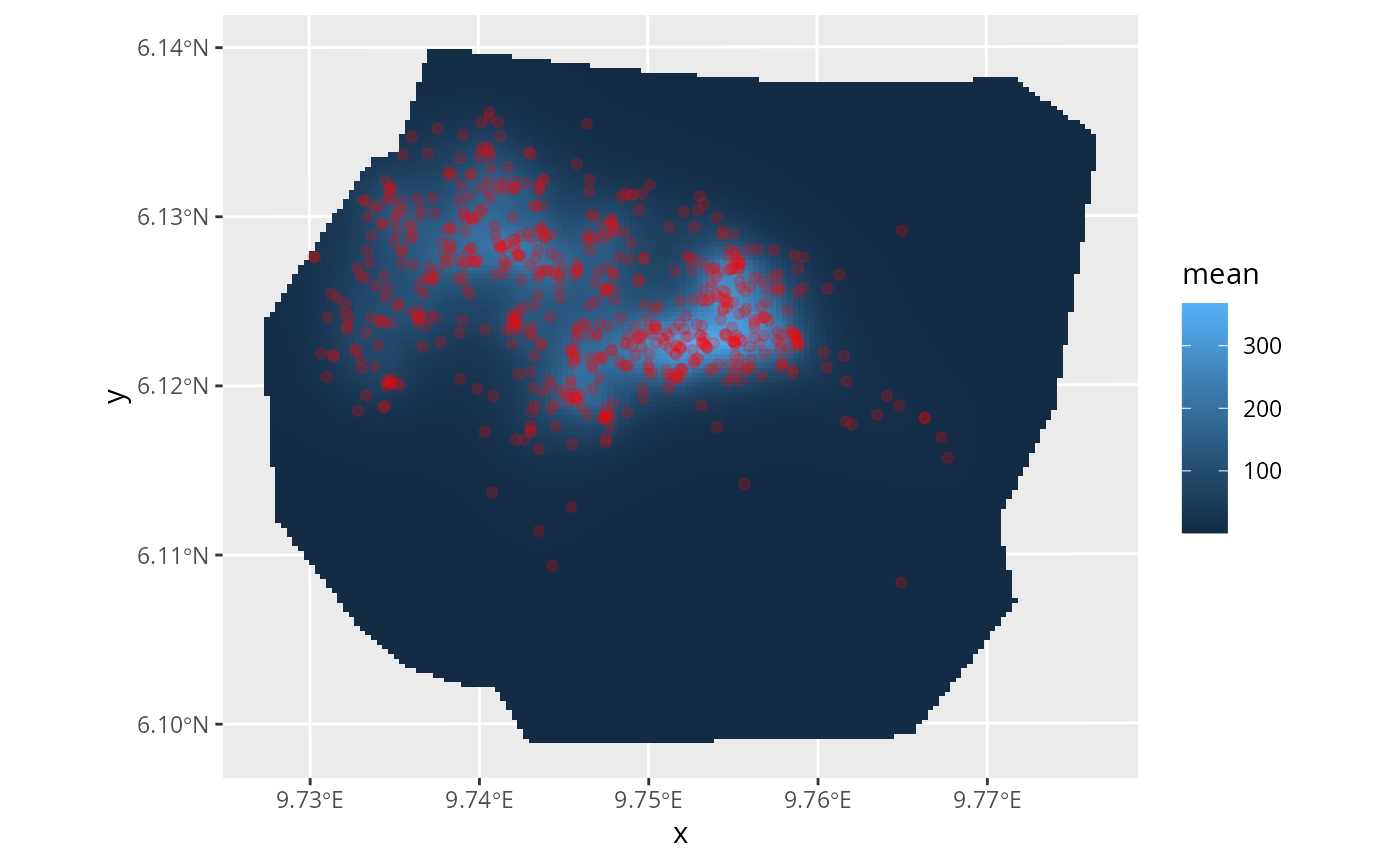This function performs inference on a LGCP observed via points residing possibly multiple dimensions. These dimensions are defined via the left hand side of the formula provided via the model parameter. The left hand side determines the intensity function that is assumed to drive the LGCP. This may include effects that lead to a thinning (filtering) of the point process. By default, the log intensity is assumed to be a linear combination of the effects defined by the formula's RHS.

More sophisticated models, e.g. non-linear thinning, can be achieved by using the predictor argument. The latter requires multiple runs of INLA for improving the required approximation of the predictor. In many applications the LGCP is only observed through subsets of the dimensions the process is living in. For example, spatial point realizations may only be known in sub-areas of the modelled space. These observed subsets of the LGCP domain are called samplers and can be provided via the respective parameter. If samplers is NULL it is assumed that all of the LGCP's dimensions have been observed completely.

## Usage

lgcp(
components,
data,
samplers = NULL,
domain = NULL,
ips = NULL,
formula = . ~ .,
...,
options = list(),
.envir = parent.frame()
)

## Arguments

components

A formula describing the latent components

data

A data frame or SpatialPoints(DataFrame) object

samplers

A data frame or Spatial[Points/Lines/Polygons]DataFrame objects

domain

Named list of domain definitions

ips

Integration points (overrides samplers)

formula

If NULL, the linear combination implied by the components is used as a predictor for the point location intensity. If a (possibly non-linear) expression is provided the respective Taylor approximation is used as a predictor. Multiple runs of INLA are then required for a better approximation of the posterior.

...

Further arguments passed on to like(). In particular, optional E, a single numeric used rescale all integration weights by a fixed factor.

options

See bru_options_set()

.envir

The evaluation environment to use for special arguments (E, Ntrials, and weights) if not found in response_data or data. Defaults to the calling environment.

## Value

An bru() object

## Examples

# \donttest{
if (bru_safe_inla() &&
require(ggplot2, quietly = TRUE) &&
require(fmesher, quietly = TRUE)) {
data <- gorillas_sf

# Plot the Gorilla nests, the mesh and the survey boundary
ggplot() +
geom_fm(data = data$mesh) + gg(data$boundary, fill = "blue", alpha = 0.2) +
gg(data$nests, col = "red", alpha = 0.2) # Define SPDE prior matern <- INLA::inla.spde2.pcmatern( data$mesh,
prior.sigma = c(0.1, 0.01),
prior.range = c(0.1, 0.01)
)

# Define domain of the LGCP as well as the model components (spatial SPDE
# effect and Intercept)
cmp <- geometry ~ field(geometry, model = matern) + Intercept(1)

# Fit the model (with int.strategy="eb" to make the example take less time)
fit <- lgcp(cmp, data$nests, samplers = data$boundary,
domain = list(geometry = data$mesh), options = list(control.inla = list(int.strategy = "eb")) ) # Predict the spatial intensity surface lambda <- predict( fit, fm_pixels(data$mesh, mask = data$boundary), ~ exp(field + Intercept) ) # Plot the intensity ggplot() + gg(lambda, geom = "tile") + geom_fm(data = data$mesh, alpha = 0, linewidth = 0.05) +
gg(data\$nests, col = "red", alpha = 0.2)
}# }